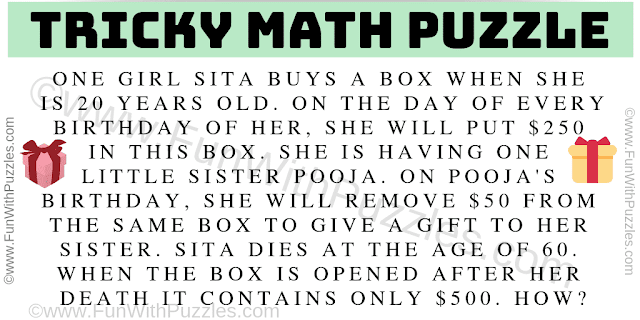This is Tricky Mathematical Puzzle which will make you think outside the box. It is a tricky math puzzle because just doing Mathematical calculations will not solve this #puzzle. One needs to think outside the box to get started with the correct maths equations which can be later solved to get the correct answer. Let us see if you can solve the tricky math puzzle?Can you solve this tricky math puzzle?
Answer of this "Tricky Math Puzzle", can be viewed by clicking on the answer button.